# Rectifier Circuits

## Discrete Semiconductor Devices and Circuits

• #### Question 1

 Don’t just sit there! Build something!!

Learning to mathematically analyze circuits requires much study and practice. Typically, students practice by working through lots of sample problems and checking their answers against those provided by the textbook or the instructor. While this is good, there is a much better way.

You will learn much more by actually building and analyzing real circuits, letting your test equipment provide the “answers” instead of a book or another person. For successful circuit-building exercises, follow these steps:

1. Carefully measure and record all component values prior to circuit construction, choosing resistor values high enough to make damage to any active components unlikely.
2. Draw the schematic diagram for the circuit to be analyzed.
3. Carefully build this circuit on a breadboard or other convenient medium.
4. Check the accuracy of the circuit’s construction, following each wire to each connection point, and verifying these elements one-by-one on the diagram.
5. Mathematically analyze the circuit, solving for all voltage and current values.
6. Carefully measure all voltages and currents, to verify the accuracy of your analysis.
7. If there are any substantial errors (greater than a few percent), carefully check your circuit’s construction against the diagram, then carefully re-calculate the values and re-measure.

When students are first learning about semiconductor devices, and are most likely to damage them by making improper connections in their circuits, I recommend they experiment with large, high-wattage components (1N4001 rectifying diodes, TO-220 or TO-3 case power transistors, etc.), and using dry-cell battery power sources rather than a benchtop power supply. This decreases the likelihood of component damage.

As usual, avoid very high and very low resistor values, to avoid measurement errors caused by meter “loading” (on the high end) and to avoid transistor burnout (on the low end). I recommend resistors between 1 kΩ and 100 kΩ.

One way you can save time and reduce the possibility of error is to begin with a very simple circuit and incrementally add components to increase its complexity after each analysis, rather than building a whole new circuit for each practice problem. Another time-saving technique is to re-use the same components in a variety of different circuit configurations. This way, you won’t have to measure any component’s value more than once.

• #### Question 2

What would this permanent-magnet DC motor do, if powered by a source of AC voltage?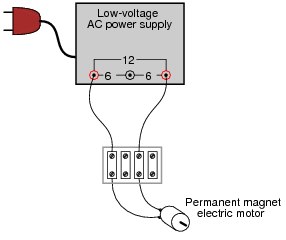• #### Question 3

What would this permanent-magnet DC motor do, if powered by the following circuit?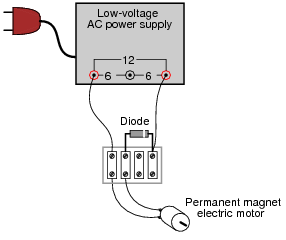• #### Question 4

What would this permanent-magnet DC motor do, if powered by the following circuit?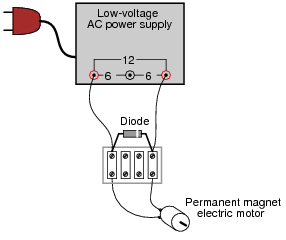• #### Question 5

Some inexpensive household lamps use a diode to achieve two-position light control (dim and bright):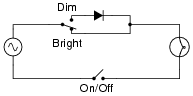Explain how this circuit is able to make the light bulb glow brighter and dimmer.

• #### Question 6

Trace the flow of all currents in this half of the AC cycle (note the polarity symbols near the transformer’s primary winding terminals):Now, trace the flow of all currents in the other half of the AC cycle (note the polarity symbols near the transformer’s primary winding terminals):Also, determine the polarity of DC voltage across the load resistor.

• #### Question 7

In this rectifier circuit, the output voltage is less than half of the secondary winding’s rated voltage (12 volts). Why is this?Also, determine whether this is a half-wave or a full-wave rectifier circuit, and explain your answer.

• #### Question 8

A very common form of full-wave rectifier circuit is the bridge rectifier. Typically, it is drawn as a “diamond” of four diodes:Draw the connections in this illustration to form a bridge rectifier circuit, receiving power from the transformer and delivering power to the light bulb: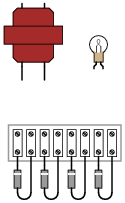• #### Question 9

Determine the approximate amount of voltage that each voltmeter in this circuit will indicate: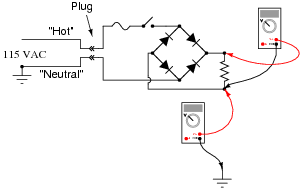• #### Question 10

A technician decides to measure the output voltage of a bridge rectifier circuit using an oscilloscope. This particular bridge rectifier is the front-end of a switching power supply circuit, and directly rectifies the incoming 120 volt AC power, with no transformer: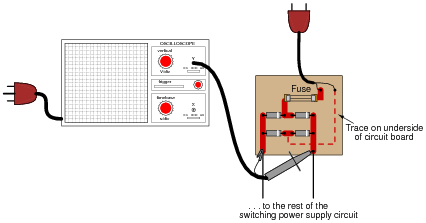However, the technician is surprised to find that the fuse blows every time she turns the power on to the circuit. When the oscilloscope is disconnected from the circuit, the fuse does not blow, and everything works fine. What is wrong? Why does the oscilloscope cause a fault in the circuit?

Here is another interesting piece of information: if just the probe tip is touched to one of the rectifier circuit’s output terminals, the oscilloscope shows a half-wave rectified waveform, without the ground clip being connected to anything!

• #### Question 11

Power rectifier circuits are often classified according to their number of phases, ways, and pulses. Match the following rectifier circuits to the Phase/Way/Pulse labels given in this illustration:• #### Question 12

Bipolar transistors are extremely useful devices, allowing a small electric current to control the flow of a much larger electric current: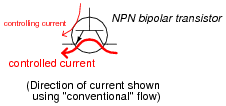These devices would be even more useful to us if they were able to control alternating current (AC), but they cannot. Bipolar transistors are polarized (one-way-only) devices.

This fact does not prevent us from using bipolar transistors to control AC. We just have to be clever about how we do it:Explain how this circuit functions. How is the transistor (a DC-only device) able to control alternating current (AC) through the load?

• #### Question 13

Suppose you need to build a full-wave bridge rectifier with a current rating of 2.5 amps, but only have model 1N4001 diodes to build it with. Draw a schematic diagram of the circuit, showing how multiple 1N4001 diodes could be connected together to accomplish this:• #### Question 14

Predict how all component voltages and currents in this circuit will be affected as a result of the following faults. Consider each fault independently (i.e. one at a time, no multiple faults):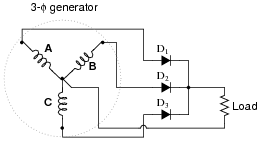Diode D1 fails open:
Generator winding C fails open:
Center connection joining generator windings fails open:

For each of these conditions, explain why the resulting effects will occur.

• #### Question 15

Predict how all component voltages and currents in this circuit will be affected as a result of the following faults. Consider each fault independently (i.e. one at a time, no multiple faults):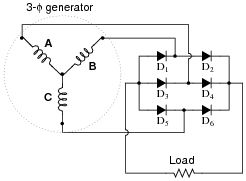Diode D3 fails open:
Generator winding C fails open:
Center connection joining generator windings fails open:

For each of these conditions, explain why the resulting effects will occur.

• #### Question 16

Trace the current through this rectifier circuit at a moment in time when the AC source’s polarity is positive on right and negative on left as shown. Be sure to designate the convention you use for current direction (conventional or electron flow):Also, mark the polarity of the voltage drop across Rload.

• #### Question 17

Trace the current through this rectifier circuit at a moment in time when the AC source’s polarity is positive on left and negative on right as shown. Be sure to designate the convention you use for current direction (conventional or electron flow):Also, mark the polarity of the voltage drop across Rload.